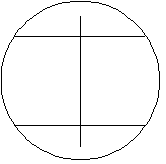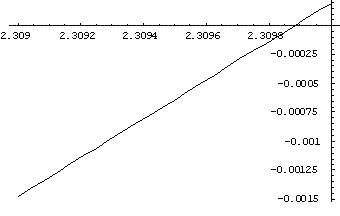#### You may also like### Baby Circle

A small circle fits between two touching circles so that all three circles touch each other and have a common tangent? What is the exact radius of the smallest circle?### Kissing

Two perpendicular lines are tangential to two identical circles that touch. What is the largest circle that can be placed in between the two lines and the two circles and how would you construct it?### Logosquares

Ten squares form regular rings either with adjacent or opposite vertices touching. Calculate the inner and outer radii of the rings that surround the squares.

# Spokes

##### Age 16 to 18Challenge Level

There are many ways to solve this problem. See the Hints for suggestions of other lines of investigation you might like to pursue to find other solutions.

Andrei from Tudor Vianu National College, Bucharest, Romania has come up with an unexpected arrangement of the sticks. Here is Andrei's solution:

 I chose to make $2$ segments of the unit, each of area a quarter of the unit circle, and the remained part of the circle to be divided into two equal parts, as shown in the figure. Let $x$ be the central angle determined by the segment. The area of the segment is: $${1\over 2}\left(x - \sin x\right).$$ And it must be a quarter the area of the circle so: $${1\over 2}\left(x - \sin x\right)= {\pi \over 4} .$$This equation could be solved only numerically, and to find an approximate solution I represent the function $x-\sin x - {\pi \over 2}$ and I look for a change of sign.To find a better approximation I used this graph. Plot $\left[x - \sin [x]- {\pi \over 2}, {x, 2.309, 2.31}\right]$ So $x \approx 2.3099$.
The length of the chord determined by the central angle $x$ in the circle of unit radius is : $$L = 2 \sin \left({x\over 2}\right) = 1.829$$ It is evident that $L$ is greater than the distance between the two parallel chords and is less than the diameter so that the problem has a solution.